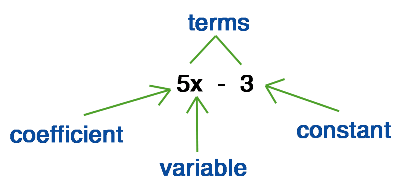Study Guide

# Basic Algebra - Algebraic Expressions

## Algebraic Expressions

Let's get down to business. An expression is made up of terms. Terms are the separate values in an expression. Each term can be a variable, a number and a variable, or a number and many variables with or without exponents, as long as everything is being multiplied together in a single nugget of math goodness. Some of our favorite terms include:

7
7x
7x2y
x6y11z2

These all count as a single term.

A number next to a variable means that number and variable are being multiplied. The same is true for two variables next to each other: it means they're being multiplied. Since the × we used for multiplication in the past looks an awful lot like the variable x, we'll stop using a symbol for multiplication at all. Some call it lazy; we call it efficient.

Some terms are numbers only. These are called constants, or numbers that don't change. Others have variables (letters that represent an unknown or changing number), and some have variables and numbers mashed together, in which case the numbers are called coefficients.

Occasionally terms, like the last one above, seem to be missing a coefficient. Here's the thing: they actually do have a coefficient of 1. It's just too lazy to show up. Since 1 times anything is just that anything, we usually don't write 1 as a coefficient, but it's always there.

Example: x is the same thing as 1x, and x3y2m is the same thing as 1x3y2m.

Variable: an unknown or changing number. Often represented by x.
Constant: a number that doesn't change.
Coefficient: the number that's multiplied by the variable.We like terms, and we especially like like terms. Like terms are terms that have the same variables, including the exponents that go with those variables. The variables can be in different orders and have different coefficients, but they all need to be there.

Examples:
3xy and -5xy are like terms (same variables).
3xy and -5xym are not like terms (the second term has a variable that the first doesn't).

-2m2xh and 4m2hx are like terms (same variables and exponents, just in different order).
-2m3xh and 4m2xh are not like terms (the variable m has different exponents in each term).

If there's more than one term separated by plus or minus signs, then we have an expression. For example:

5x2y – 3xy + y + 5

This expression has four terms: 5x2y, -3xy, y (or 1y), and 5.

### Names for algebraic expressions

 monomial 1 Term xy binomial 2 Terms xy – 2x trinomial 3 Terms xy – 2x + 3y quadnomial 4 Terms xy – 2x + 3y – 1

Here is a chart of common phrases.

Common Words and Phrases for:
AddSubtractMultiplyDivideEquals
plus
add
sum
more than
in addition to
greater than
total
and
difference
subtract
less than
take away
product
of
times
twice (×2)
factor
divided by
quotient
split
share
distribute
is

In these translations, we'll use the letter x to represent the variable, though any letter, symbol, or emoticon would work. Smiley faces and hearts, anyone?

Expression in WordsExpression in Symbols
a number increased by twelvex + 12
the sum of twice a number and six2x + 6
eighty less than a numberx – 80
twenty-eight split in half28 ÷ 2
the product of a number and seven7x
the quotient of a number and fourx ÷ 4
five greater than three times a number3x + 5
a number distributed evenly among sixx ÷ 6
the total of forty and a number40 + x
three times the total of a number and five3(x + 5)
Equation in WordsEquation in Symbols
A number is negative ten.x = -10
A number plus two is eight.x + 2 = 8
The difference between a number and seven is negative three.x – 7 = -3
One less than twice a number is seventeen.2x – 1 = 17
Twelve is the product of a number and three.12 = 3x
Half of a number is twenty.½x = 20
A number is equal to the sum of twice the number and negative three.x = 2x + (-3)

Look Out: be very careful with "less than." Three less than a number is translated as "x – 3." The reverse of that, "3 – x," would be a number less than 3.